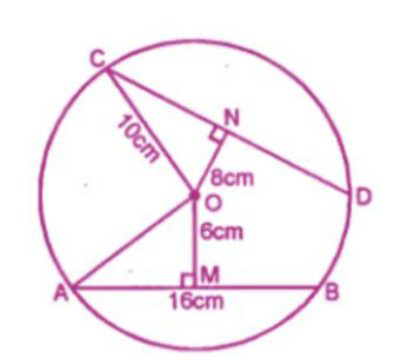# ML Aggarwal Solutions Class 9 Mathematics Solutions for Circle Exercise 15.1 in Chapter 15 - Circle

A chord of length 16 cm is at a distance of 6 cm from the centre of the circle. Find

the length of the chord of the same circle which is at a distance of 8 cm from the

centre.

AB is a chord a circle with center O and

OA is the radius of the circle and OM ⊥ ABAB = 16 cm, OM = 6 cm

OM ⊥ AB

AM = ½ AB = ½ × 16 = 8 cm

Now in right ∆OAM

\begin{aligned} &\mathrm{OA}^{2}=\mathrm{AM}^{2}+\mathrm{OM}^{2}\\ &\text { (By Pythagoras Axiom) }\\ &=(8)^{2}+(6)^{2}\\ &64+36=100=(10)^{2} \end{aligned}

Now CD is another chord of the same circle

ON ⊥ CD and OC is the radius.

In right ∆ONC

\begin{aligned} &\mathrm{OC}^{2}=\mathrm{ON}^{2}+\mathrm{NC}^{2}\\ &\text { (By Pythagoras Axioms) }\\ &(10)^{2}=(8)^{2}+(N C)^{2}\\ &100=64+\mathrm{NC}^{2}\\ &\mathrm{NC}^{2}=100-64=36=(6)^{2} \end{aligned}

NC = 6

But ON ⊥ AB

N is the mid-point of CD

CD = 2 NC = 2 × 6 = 12 cm

Video transcript
"hello and welcome idea students i'm surprised you don't know leader and i'm here the new question it is a chord of length 16 centimeter is at a distance of 6 centimeter from the center of this circle find the length of the chord of the same circle which is at a distance of 8 centimeter from the center so without wasting much time let's have a look at the solution so we know that a b is required of the circle at center o and o is the radius of the circle and also om is perpendicular to a b we know that a b is 16 centimeter and om is six centimeter since we know that om is perpendicular to a b so this implies that am is half of a b which is nothing but half of 16. nothing but equals to 8 centimeter so therefore in triangle om four a square is equals to om square plus am square which further implies that osb is equals to om square which is 6 which is nothing but equal to 36 whereas am square am is 8 am square is 64 so which means that 2a square is 100 and oa is nothing but equals to 10 centimeter and since we know that o and oc are equal because both these are the radius of the same circle so oc is also 10 centimeter now cd is another chord of the same circle where o n is perpendicular to c d and o c is the radius so in right triangle i am writing rt for right o nc we get cozy square which is equals to on square plus cn square which further implies that oc is 10 so this is 10 square o n is 8 so 8 square plus c n square which further implies that c n is equals to root over 100 minus 64 which is nothing but equal to root over which is nothing but equals to six centimeter and since we know that so you know but o n is perpendicular to c d which implies that n is the midpoint of c d therefore cd is equals to twice of cn which further implies that cd is equals to twice of six which is equal to 12 centimeter so i hope my students got this question well do subscribe our channel for the regular updates you can also share your doubt in the comment section see you next time bye"
Related Questions

Lido

Courses

Teachers

Book a Demo with us

Syllabus

Maths
CBSE
Maths
ICSE
Science
CBSE

Science
ICSE
English
CBSE
English
ICSE
Coding

Terms & Policies

Selina Question Bank

Maths
Physics
Biology

Allied Question Bank

Chemistry
Connect with us on social media!